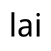# From a mathematical point of view, whether it is "artificial intelligence" or "artificial intellectual disability"

author：Daily chatgpt

Looking at mathematical problems today, for ChatGPT and Wen Xin, the dimension of thinking about mathematical problems determines whether they can accurately understand and calculate the results.

Problem: Chickens, ducks and rabbits have a total of 6 heads and 14 legs, and the chicken has one more chicken than a duck. Ask how many chickens, ducks and rabbits there are.

This is a question that tests the dimension of AI thinking, first to understand the topic, and second to knowFrom a mathematical point of view, whether it is "artificial intelligence" or "artificial intellectual disability"

The training dimension of AI can currently reach 100 million+

Attributes of chickens, ducks and rabbits: head, legs.

Third, know how the results are calculated based on their relationship.

Let's talk about the conclusion: GPT3.5 and Wen Xin did not give the correct result, GPT-4.0 gave the correct result.

Take a look at Wen Xin's words:

Wen Xin said

GPT3.5

It can be seen that Wen Xin's words have improved compared with the previous chicken and rabbit in the same cage, but the shortcomings are very obvious: it is calculated by breaking fingers, so it is impossible to calculate correctly.

GPT-3.5 is much better, although it is not correct, but the idea of equations is a huge improvement, with equations can solve more complex problems. But unfortunately, the last step of the equation is still wrong, and the dimension of thinking in this regard is still a little less.

I have highlighted the solution process of GPT-4.0.

We can solve this problem using algebraic methods. Let the number of chickens be x, the number of ducks y, and the number of rabbits z. Depending on the topic, we can get the following equation:

1. x + y + z = 6 (number of headers)
2. 2x + 2y + 4z = 14 (number of legs)
3. x = y + 1 (one more chicken than duck)

We can first solve the first and third equations to get the relationship between y and z:

y + z = 5 - x y = x - 1

Substituting the third equation into the first yields:

x - 1 + z = 5 - x 2x = 6 x = 3

So the number of chickens is 3. Substituting the value of x into the third equation yields:

y = 3 - 1 y = 2

So the number of ducks is 2. Finally, substituting the values of x and y into the first equation yields:

3 + 2 + z = 6 z = 1

So the number of rabbits is 1.

To sum up, there are 3 chickens, 2 ducks and 1 rabbit.

Therefore, which model is "intelligent" or "retarded" depends on its thinking dimension, although we do not know how they are trained. But the result is verifiable.

At present, Wen Xin is still a primary school student compared to ChatGPT, 3.5 in ChatGPT is a junior high school student, and 4.0 is a college student. The gap is so obvious!

10-01

10-01

10-01

10-01

10-02

10-02

10-02

10-02

10-02

10-02

10-02

10-02

10-02

10-02

10-02

10-02